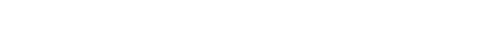glBegin.3glOpenGL man pages

```

```

## Name

```  glBegin, glEnd - delimit the vertices	of a primitive or a group of like
primitives

```

## C Specification

```  void glBegin(	GLenum mode )

```

## Parameters

```
mode	Specifies the primitive	or primitives that will	be created from
vertices presented between glBegin and the subsequent glEnd.  Ten
symbolic constants are accepted: GL_POINTS, GL_LINES, GL_LINE_STRIP,
GL_LINE_LOOP, GL_TRIANGLES, GL_TRIANGLE_STRIP, GL_TRIANGLE_FAN,

```

## C Specification

```  void glEnd( void )

```

## Description

```  glBegin and glEnd delimit the	vertices that define a primitive or a group
of like primitives.  glBegin accepts a single	argument that specifies	which
of ten ways the vertices are interpreted.  Taking n as an integer count
starting at one, and N as the	total number of	vertices specified, the
interpretations are as follows:

GL_POINTS Treats each	vertex as a single point.  Vertex n defines point n.
N points are drawn.

GL_LINES  Treates each pair of vertices as an	independent line segment.
Vertices 2n-1 and 2n define	line n.	 N/2 lines are drawn.

GL_LINE_STRIP
Draws a connected group of line segments from the first vertex to
the	last.  Vertices	n and n+1 define line n.  N-1 lines are
drawn.

GL_LINE_LOOP
Draws a connected group of line segments from the first vertex to
the	last, then back	to the first.  Vertices	n and n+1 define line
n.	The last line, however,	is defined by vertices N and 1.	 N
lines are drawn.

GL_TRIANGLES
Treates each triplet of vertices as	an independent triangle.
Vertices 3n-2, 3n-1, and 3n	define triangle	n.  N/3	triangles are
drawn.

GL_TRIANGLE_STRIP
Draws a connected group of triangles.  One triangle	is defined
for	each vertex presented after the	first two vertices.  For odd
n, vertices	n, n+1,	and n+2	define triangle	n.  For	even n,
vertices n+1, n, and n+2 define triangle n.	 N-2 triangles are
drawn.

GL_TRIANGLE_FAN
Draws a connected group of triangles.  One triangle	is defined
for	each vertex presented after the	first two vertices.  Vertices
1, n+1, and	n+2 define triangle n.	N-2 triangles are drawn.

GL_QUADS  Treats each	group of four vertices as an independent
quadrilateral.  Vertices 4n-3, 4n-2, 4n-1, and 4n define

defined for	each pair of vertices presented	after the first	pair.
Vertices 2n-1, 2n, 2n+2, and 2n+1 define quadrilateral n.  N/2-1
quadrilaterals are drawn.  Note that the order in which vertices
are	used to	construct a quadrilateral from strip data is
different from that	used with independent data.

GL_POLYGON
Draws a single, convex polygon.  Vertices 1	through	N define this
polygon.

Only a subset	of GL commands can be used between glBegin and glEnd.  The
commands are glVertex, glColor, glIndex, glNormal, glTexCoord, glEvalCoord,
glEvalPoint, glMaterial, and glEdgeFlag.  Also, it is	acceptable to use
glCallList or	glCallLists to execute display lists that include only the
preceding commands.  If any other GL command is executed between glBegin
and glEnd, the error flag is set and the command is ignored.

Regardless of	the value chosen for mode, there is no limit to	the number of
vertices that	can be defined between glBegin and glEnd.  Lines, triangles,
quadrilaterals, and polygons that are	incompletely specified are not drawn.
Incomplete specification results when	either too few vertices	are provided
to specify even a single primitive or	when an	incorrect multiple of
vertices is specified. The incomplete	primitive is ignored; the rest are
drawn.

The minimum specification of vertices	for each primitive is as follows: 1
for a	point, 2 for a line, 3 for a triangle, 4 for a quadrilateral, and 3
for a	polygon.  Modes	that require a certain multiple	of vertices are

```

## Errors

```  GL_INVALID_ENUM is generated if mode is set to an unaccepted value.

GL_INVALID_OPERATION is generated if a command other than glVertex,
glColor, glIndex, glNormal, glTexCoord, glEvalCoord, glEvalPoint,
glMaterial, glEdgeFlag, glCallList, or glCallLists is	executed between
glBegin and the corresponding	glEnd.

GL_INVALID_OPERATION is generated if a glBegin is executed between a
glBegin and the corresponding	execution of glEnd.

GL_INVALID_OPERATION is generated if glEnd is	executed without being
preceded by a	glBegin.

```

```  glCallList, glCallLists, glColor, glEdgeFlag,	glEvalCoord, glEvalPoint,